MATrix LABoratory (MATLAB) is developed by The Mathworks, Inc. It is an interactive, integrated environment for numerical scientific computations and visualization, as well as symbolic computations, and others. MATLAB is known especially for its highly intuitive and interactive programming development and debugging environment which results in a relatively short development cycle compared with traditional compiled languages such as FORTRAN and C.

### Getting Started with MATLAB

• To start MATLAB in Windows: double click the desktop MATLAB icon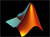• To start MATLAB in Linux: enter `matlab` at the system prompt of a X-Forwarding window
``scc1% matlab &``

In either case, a MATLAB command window appears, along with a `>>` prompt. Start using MATLAB by entering MATLAB commands at `>>`. The example below demonstrates the use of a MATLAB utility function called `version` to query the current version of MATLAB you are using on this computer. Note that all typed characters to the right of the `%` symbol are treated as explanations or documentation for the line. Output, if any, appears after the the command is entered.

``````>> version   % this tells you the running MATLAB version

ans =

8.1.0.604 (R2013a)``````

Throughout this tutorial, we will use this (`%`) inline comment feature to explain the meaning or purposes of the commands. When you are ready to start writing your own code, you may want to take advantage of this to document your own code wherever appropriate to make clear your intentions for future reference.

``````>> help         % lists available packages/toolboxes.
HELP topics:

.  .  .  .  .  .
matlab/general     - General purpose commands.
matlab/ops         - Operators and special characters.
matlab/lang        - Programming language constructs.
matlab/elmat       - Elementary matrices and matrix manipulation.
matlab/randfun     - Random matrices and random streams.
matlab/elfun       - Elementary math functions.
.  .  .  .  .  .
.  .  .  .  .  .

>> help elfun      % lists elementary math functions
Elementary math functions.

Trigonometric.
sin         - Sine.
sind        - Sine of argument in degrees.
.   .   .   .

>> help sin      % if you know function name but not it's usage
SIN    Sine of argument in radians.
SIN(X) is the sine of the elements of X.
.   .   .

>> lookfor cosine    % if you don't know the function name
acos                      - Inverse cosine, result in radians.
acosd                     - Inverse cosine, result in degrees.
acosh                     - Inverse hyperbolic cosine.
cos                       - Cosine of argument in radians.
.   .   .

>> doc sin     % spawns a new document window with details on sin>> quit        % quits MATLAB (also exit)``````

### Rules on Variable Names

• Names are case sensitive, e.g., NAME and Name are 2 distinct names.
• Variable names begin with a letter, e.g., A2z or a2z. On the other hand, 2Az is not valid
• Names can be a mix of letters, digits, and underscores (e.g., vector_A)
• Cannot use reserved characters:    %    =    +   .   ~   ;   :     !     ‘     [   ]   (   )   ,   @     #     \$   &     ^
• Names can be up to 63 characters

### Reserved Characters   %   =   ;   ,

Some characters are reserved by MATLAB for various purposes. Some are arithmetic or matrix operators:

=,  +,  – ,  *,  / ,  and others are used to perform a multitude of operations. Reserved characters can not be used in variable, function, or file names.

``````>> % text after % until end of line treated as comments
>>
>> a = 3     % define a to have the value 3

a =
3

>> a = 3; %  ;  suppresses printing
>>
>> b = 4; c = 5;  % ;  delimits multiple commands on same line
>>
>> d = 6, e = 7;  % ,  delimits commands but enables printing

d =
6
>> a#2=3   % use of reserved character in variable name is illegal
``````
??? a#2=3 | Error: The input character is not valid in MATLAB statements or expressions.

### Reserved Characters    :   [   ]   (  )

``````>> x = 1:2:9   % define vector x with : operator (begin:interval:end)

x =
1     3     5     7     9

>> y = 3:5   % interval is defaulted to 1; same as y = 3:1:5

y =
3     4     5

>> X = [1, 2, 3; 4, 5, 6]  % 2D array.
% [ ] prevents ambiguity for arrays
% ; concatenates vertically (new row)
% , concatenates horizontally  (new columns)

X =
1     2     3
4     5     6``````

Form “composite” string with brackets

``````>> n=12; S = ['This string consists of characters & numbers: ' num2str(n)]
S =
This string consists of characters and numbers like: 12

In the above, num2str must be used to convert n, a double, to string for
data type consistency. To print the content of S more cleanly
>> disp(S)    % or replace S with the string it represents
This string consists of characters and numbers like: 12

More generally, use sprintf
>> help sprintf  % for details; %d is format for integers; %f for decimal numbers
>> str = sprintf('This string consists of characters & numbers: %d\n', n);

Likewise, to just print a message
>> disp(sprintf('This string consists of characters & numbers: %d\n', n))``````
Parentheses, on the other hand, are used to specify the order of operations and express a statement more clearly. They are also used to refer to an element of a matrix.
``````>> a = 3; y = 1 + (a+5)/4;
>> X(2,3)     % ( ) for subscripting;  why ans ?

ans =
6``````

### Reserved Characters    …   and    ‘

``````>> x = [1 2 3 ...   % to be continued
4 5 6]

x =

1     2     3     4     5     6

>> s = 'this is a character string';  % blanks preserved within quotes
>> x = [1 2 3]'    % transposes (in this case, turns row into column)

x =
1
2
3

>> X = [1 2 3; 4 5 6]; size(X)   % size (dimensions) of X

ans =
2     3

>> X = [1 2 3; 4 5 6]; numel(X)  % number of elements in X

ans =
6``````

### Reserved Character    ! (or system, perl, java, python)

On occasions, there might be a need to perform tasks on the host system and return the output back to the MATLAB space. For this, you can enter the host system-based command, preceded with a !, at the MATLAB prompt.
``````>> !dir     % runs a dos command on Windows host
Volume in drive C has no label.
Volume Serial Number is 6860-EA46
Directory of C:Program FilesMATLAB704work
01/31/2007  10:56 AM               .
01/31/2007  10:56 AM               ..
06/13/2006  12:09 PM                12 foo.exe
06/13/2006  08:57 AM                77 mkcopy.m

>> !ls -l   % runs a unix command on Linux host
total 0
-rw-r--r--  1 kadin scv 0 Jan 19 15:53 file1.m
-rw-r--r--  1 kadin scv 0 Jan 19 15:53 file2.m
-rw-r--r--  1 kadin scv 0 Jan 19 15:53 file3.m
>> system('ls  -l')   % more general form; also unix('ls  -l')``````

### Array operations

``````>> a = 1:3;        % a is a row vector
>> b = 4:6;        % b is a row vector
>> c = a + b       % a & b must have same shape & size; c has same shape as a & b
c =
5     7     9
>> d = a(1:2) + b(2:3)  % array sections must conform
d =
6     8

>> A = [a;b]   % combines rows to generate 2x3 matrix A
% what is the outcome of A=a;b  ?
A =
1     2     3
4     5     6

>> B = A'    % B is transpose of A
B =
1     4
2     5
3     6``````

Other ways to create B ? (hint: with a and b )

### Matrix Operations

One of the many nice features of MATLAB is its ability to perform operations based on the properties of the operands. For instance, if a and b are scalars, then c = a * b is a scalar. However, if A and B are matrices, then * will be treated as a matrix multiply operator. As a result, the number of columns of A must match the number of rows of B.
``````>> C = A*B    % * is overloaded  as matrix multiply operator
% i.e., Cij = &#931k Aik*Bkj; for all i and j; sum over index k
C =
14    32
32    77

>> D = A.*A   % .* turns matrix multiply to elemental multiply
% i.e., Dij = Aij*Aij; for all i and j
D =
1     4     9
16    25    36

>> E = A./A   % elemental divide; Eij = Aij/Aij
E =
1     1     1
1     1     1

>> who        % list existing variables in workspace
A    B    C    D    E    a    b    d``````

### Data Types

In many languages, programmers are required to declare variable data types, such as integer, float, character, and so on. In MATLAB, programmers are not required to declare the data types of variables. In MATLAB, the only default underlying data types are double and character. Essentially, a variable that is not defined between a pair of single quotes, such as a = ‘this is a string’ is considered a double precision number.
``````>> whos     % detail listing of workspace variables
Name         Size          Bytes  Class
A         2x3              48  double array
B         3x2              48  double array
C         2x2              32  double array
D         2x3              48  double array
E         2x3              48  double array
a         1x3              24  double array
b         1x3              24  double array
c         1x3              24  double array
Grand total is 37 elements using 296 bytes

>> A = single(A);    % recast A to single data type to save memory
>> whos
Name         Size         Bytes  Class
A          2x3            24  single array
.   .   .   .

>> clear  % delete all workspace variables``````

### For Loops

``````for k=1:5   % use for-loops to execute iterations / repetitions
for j=1:3
for i=1:4
a(i, j, k) = i + j + k;
end
end
end``````

Utilities to initialize or define arrays: `ones, rand, eye, . . .`

Trigonometric and hyperbolic functions : `sin, cos, sqrt, exp, . . .`

These utilities can be used on scalar or vector inputs. For example,

``>> a = sqrt(5); v = [1 2 3]; A = sqrt(v);``

### if Conditional

Scalar operation . . .

``````for j=1:3
for i=1:3
a(i,j) = rand;    % use rand to generate a random number
b(i,j) = 0;
if a(i,j) > 0.5
b(i,j) = 2;    % set b(i,j) to 2 whenever the condition a(i,j) > 0.5 is satisfied
end
end
end``````

Equivalent vector operations . . .

``````A = rand(3);      % A is a 3x3 random number double array
B = zeros(3);     % Initialize B as a 3x3 array of zeroes
B(A > 0.5) = 2;   % for all A(i,j) > 0.5, set B(i,j) to 2``````

Note that the long form of the above expression is

``````L = A > 0.5;  % L is logical array; L(i,j) = 1 for all A(i,j) > 0.5; 0 otherwise
B(L) = 2      % B(i,j) = 2 whenever L(i,j) = 1  (i.e., true)``````

Elemental Matrix Division
The purpose is to compute elemental division with the denominator matrix having zeroes. For those situations, `c` is to be set to 0.

Scalar form . . .

``````a = rand(4,3); b = rand(size(a)); c = zeros(size(b));
b(1,3) = 0; b(3,2) = 0;   % reset 2 specific elements of b to 0
for j=1:3
for i=1:4
if (b(i,j) ~= 0) then   % if b(i,j) not equals 0
c(i,j) = a(i,j)/b(i,j);
end
end
end``````

The equivalent vector operation . . .

``````c(b~=0) = a(b~=0) ./ b(b~=0);   % define c=a./b for all b &#8800 0
% b~=0 needed everywhere to ensure matched array shape/size``````

### Logical Arrays

Alternatively, the above example may be performed with the help of an explit logical array

``````e = b~=0    % e is logical array, true(1) for all b ≠ 0 (zero rather than logical false)
e =

1      1      0
1      1      1
1      0      1
1      1      1

c(e) = a(e)./b(e)   % c = 0  ∀  b = 0, else c=a./b
c =

0.9768    1.4940         0
2.3896    0.4487    0.0943
0.7821         0    0.2180
11.3867    0.0400    1.2741``````

In MATLAB, a number divided by 0 returns an Inf rather than “division by zero” and crashed ! Hence, an alternative way to handle the above conditional computation is

```>> c = a ./ b   % elemental divide
c =

0.9768    1.4940       Inf
2.3896    0.4487    0.0943
0.7821       Inf    0.2180
11.3867    0.0400    1.2741

Followed by
>> c(c == Inf) = 0    % whenever c(i,j) equals Inf, reset it to 0
c =

0.9768    1.4940         0
2.3896    0.4487    0.0943
0.7821         0    0.2180
11.3867    0.0400    1.2741```

### Cell Arrays

A cell array is a special array of arrays. Each element of a cell array may point to a scalar, an array, or another cell array. Unlike a regular array, the elements of a cell array need not be uniformly of a single data type.

``````>> C = cell(2, 3);   % create 2x3 empty cell array
>> M = magic(2);
>> a = 1:3; b = [4;5;6]; s = 'This is a string.';
>> C{1,1} = M; C{1,2} = a; C{2,1} = b; C{2,2} = s; C{1,3} = {1};
C =
[2x2 double]      [1x3 double]         {1x1 cell}
[2x1 double]      'This is a string.'    []
>> C{1,1}     % prints contents of a specific cell element
ans =
1     3
4     2
>> C(1,:)     % prints first row of cell array C; not its content``````

Related utilities: `iscell`, `cell2mat`

### Structures

Ideal layout for grouping arrays that are related.

``````>> employee(1).last = 'Smith';  employee(2).last  = 'Hess';
>> employee(1).first = 'Mary';  employee(2).first = 'Robert';
>> employee(1).sex = 'female';  employee(2).sex = 'male';
>> employee(1).age = 45;        employee(2).age = 50;
>> employee(2)    % list contents of employee 2
ans =
last: 'Hess'
first: 'Robert'
sex: 'male'
age: 50
>> employee(:).last     % display last name of all employees
ans =
Smith
ans =
Hess``````

To avoid seeing “ans”, you can save the content to a variable first. Note however that because the last names vary in byte size, the variable should be a cell array.

``````>> a = {employee(:).last}    % a = employee(:).last would fail
a =

'Smith'    'Hess'``````

Alternative style:

``````>> employee = struct('last',{'Smith','Hess'}, 'first',{'Mary','Robert'},
'sex',{'female','male'}, 'age',{45,50});``````

Related utilities: `isstruct`, `fieldnames`, `getfield`, `isfield`

### File Types

There are many types of files in MATLAB :

• script m-files (.m) — a collection of related commands towards an objective; when script is invoked in a workspace, the commands in script are executed in order in that space; memory access is transparent because the caller (where script is invoked) and callee (the script) share the same workspace
• function m-files (.m) — an insulated form of script; memory access is controlled: variables’ content needed must be passed as input while output from the function must be explicitly returned to caller
• mat files (.mat) — binary (or text) files handled with save and load
• mex files (.mex) — files enabling calling C/FORTRAN codes from m-file
• eng files (.eng) — files enabling calling m-file from C/FORTRAN codes
• C codes (.c) . C codes generated by MATLAB compiler
• P codes (.p) . converted m-files to preserve source code privacy

### Script m-file

For operations that are not exploratory in nature or requiring more than a handful of commands to accomplish, it is often more practical to save the operational procedure into an m-file, which is a file with .m suffix. Changes to the procedure can be made more easily with a file than re-typing from scratch. To run it, just enter the file name at the `>>` prompt without `.m`. The commands in the file are executed in turn. This m-file can be reused such as in a loop, in multiple sections of an application, or in different applications. We will demonstrate the process of creating and running a script below. While you may use any editor, here is how you can create a file `myMean.m` with the MATLAB editor. The MATLAB editor is an interactive development environment: it is an editor; a debugger; programming spell checker; you can even run code within it.

``````>> edit myMean.m   % invoke MATLAB editor; type the following commands in editor window
% myMean.m
% Computes the arithmetic mean of x
% x      (input) matrix for which the arithmetic mean is sought
% means (output) the average of x  ( = [x(1)+ x(2) + . . . + x(n)]/n )
% MATLAB equivalent utility is mean
means = sum(x)/numel(x);    % the arithmetic mean``````

Select Save from the Editor window’s Menu bar to save it as `myMean.m`. Shown below is an example of the run procedure

``````>> clear   % clear base workspace
>> whos    % list content of workspace
>>
>> x=1:3;  % define a vector x=[1 2 3]
>> myMean; % x is accessible to myMean.m as both share the same MATLAB workspace
>> whos    % list content of workspace
Name       Size            Bytes  Class     Attributes
means      1x1                 8  double
x          1x3                24  double

>> means   % means = (1 + 2 + 3)/3 = 2
means =
2
``````

#### Notes on script m-files

• On the one hand, a script m-file blends seamlessly with the workspace where it was launched. On the other hand, because of the common memory space, there could be unintentional consequences if care is not exercised. Let say that you have a variable `x` in the current workspace. Any script m-file launched in this workspace has full access to `x`. If you redefine or delete `x` within this script m-file, `x` will be changed or removed.
• In the example above, `x` is not defined inside the script to enable wider range.
• Script m-files are not the best tool for repeated usage such as inside a loop; it may be unsafe (see above) and it is computationally inefficient compared with an equivalent function m-file.

### Function m-files

• A function m-file must be declared with the keyword `function`
• A function is insulated. It lives in its own workspace. All input and output variables to the function must be passed between the workspace from which the function is invoked and the function’s own workspace.

As an example, use MATLAB editor to create a file that computes the average of a set of numbers:

``````>> edit average.m

function avg=average(x)
% function avg=average(x)
% Computes the arithmetic mean of x
% x      (input) matrix for which the arithmetic mean is sought
% avg   (output) the average of x  ( = [x(1)+ x(2) + . . . + x(n)]/n )
% MATLAB equivalent utility is mean
avg = sum(x)/numel(x);    % the average
end``````

Save the above with `File/Save`

While not required, it is recommended to save file by the name of the function to avoid confusions. In the above, average is the function name and the file is saved as `average.m`. All input parameters are passed into the function as arguments on the right of the equal sign (`=`) while the optional output appear on the left. If you have multiple output, they should be enclosed with brackets, e.g., `[a, b, c]`.

It may be called from a script, another function, or from the command line:

``````>> a = average(1:3)    % a = (1 + 2 + 3) / 3
a =
2
>> help average        % prints contiguous lines that starts with % at top of average.m``````

#### Notes on function m-files

• All variables in a function are effectively local variables.
Temporary variables allocated inside the function are local. Input variables on the function declaration are copied from the caller, known as “passed by copy.” On the contrary, output variables are local until they are copied back to the caller when returned. Exceptions: `global` variables are not copied; input variables that are used “as is,” i.e., with no changes, are their corresponding variables on the caller to avoid copying, known as “passed by reference.” MATLAB calls this lazy copy.

``````>> b = 99;          % define b
>> y = 1:3;         % y is "lazy-copied" into average as x
>> b = average(y)   % output a of average copied to b on caller; replaces "old" b
b =
2``````
• The above leads to a well insulated environment which enables a function to be developed, practically independent from, and without much consideration for, the caller environment. This is one of the key features that make function m-files preferred over script m-file.
• Computationally a function m-file is more efficient than a script m-file. When a function is invoked for the first time in a MATLAB session, it is compiled into a pseudo-code. Subsequent execution of the function (now pre-compiled) will be more efficient. If it is used inside a large loop, the saving could be very significant. A script m-file is never compiled and will not benefit from repeated usage.

• It is common for MATLAB utilities or users’ own m-files to be overloaded. For example, someone using your function may pass an input variable x as scalar, vector, or matrix. Can your function handle that ? It is a good practice to document your function’s usage rules in the documentation section of the function (contiguous lines that start with % at the top).
``````>> y = [1:3;4:6]    % y is now a 2x3 array
y =

1     2     3
4     5     6``````

First, we use the MATLAB arithmetic mean utility to compute the means of y.

``````>> a = mean(y)      % mean computes means for each column of y
a =

2.5000    3.5000    4.5000

>> b = average(y)   % means of y with average is wrong; use of numel not suitable
b =

0.8333    1.1667    1.5000``````

The computed results of average are wrong! That’s because the use of `numel` is not appropriate for multi-dimensional `y`. How would you fix it ?

A closer look at the function `average` reveals that it requires a `sum` which, when applied to say a 2-dimensional array, computes column sums. With that awareness and the use of the colon operator, we could force `y` to be a vector with `y(:)`. When used on `sum`, it yields a global sum. Similarly,

``````>> c = mean(y(:))   % more compact and efficient than mean(mean(y))
c =

3.5000
``````

### Some Frequently Used Functions

``````>> magic(n);     % creates a special n x n  matrix; handy for testing
>> zeros(n,m);   % creates n x m matrix of zeroes (0)
>> ones(n,m);    % creates n x m matrix of ones (1)
>> rand(n,m);    % creates n x m matrix of random numbers
>> repmat(a,n,m);% replicates a by n rows and m columns
>> diag(M);      % extracts the diagonals of a matrix M
>> help elmat    % list all elementary matrix operations (or elfun)
>> abs(x);       % absolute value of x
>> exp(x);       % e to the x-th power
>> fix(x);       % rounds x to integer towards 0
>> log10(x);     % common logarithm of x in base 10
>> rem(x,y);     % remainder of x/y
>> mod(x, y);    % modulus after division; unsigned rem
>> sqrt(x);      % square root of x
>> sin(x);       % sine of x; x in radians
>> acoth(x)      % inversion hyperbolic cotangent of x``````

### MATLAB Graphics

• Line plot
• Bar graph
• Surface plot
• Contour plot
• MATLAB tutorial on 2D, 3D visualization tools as well as other graphics packages available in our tutorial series.

### Line Plot

``````>> t = 0:pi/100:2*pi;
>> y = sin(t);
>> plot(t,y)``````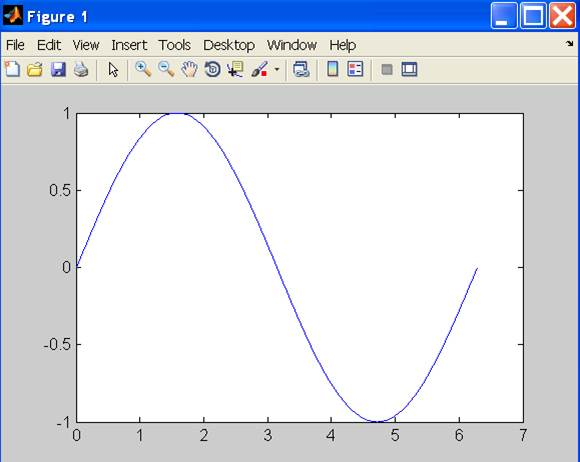### Line Plot – continues

``````>> xlabel('t');
>> ylabel('sin(t)');
>> title('The plot of t vs sin(t)');``````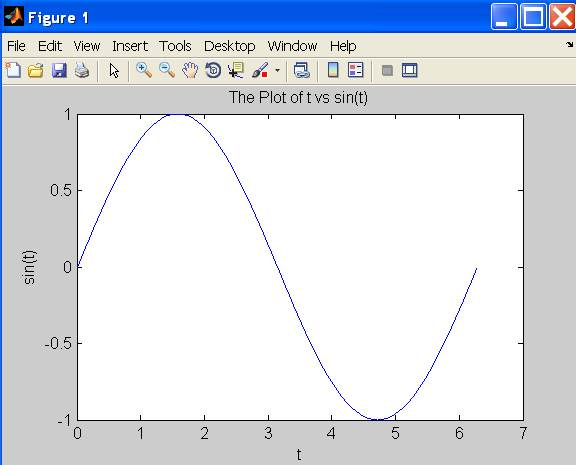### Line Plot – continues

``````>> y2 = sin(t-0.25);
>> y3 = sin(t+0.25);
>> plot(t,y,t,y2,t,y3)   % make 2D line plot of 3 curves
>> legend('sin(t)','sin(t-0.25)','sin(t+0.25',1)``````### Customizing Graphical Effects

Generally, MATLAB’s default graphical settings are adequate which makes plotting fairly effortless. For more customized effects, use the get and set commands to change the behavior of specific rendering properties.

``````>>> hp1 = plot(1:5)   % returns the handle of this line plot
>> get(hp1)    % to view line plot's properties and their values
>> set(hp1, 'lineWidth')   % show possible values for lineWidth
>> set(hp1, 'lineWidth', 2)     % change line width of plot to 2
>> gcf             % returns current figure handle
>> gca            % returns current axes handle
>> get(gcf)      % gets current figure's property settings
>> set(gcf, 'Name', 'My First Plot')   % Figure 1 => Figure 1: My First Plot
>> get(gca)     % gets the current axes.  property settings
>> figure(1)     % create/switch to Figure 1 or pop Figure 1 to the front
>> clf               % clears current figure
>> close          % close current figure; "close 3" closes Figure 3
>> close all     % close all figures``````

### 2D Bar Graph

``````>> x = magic(3);    % generate data for bar graph
>> bar(x)               % create bar chart
>> grid                  % add grid for clarity``````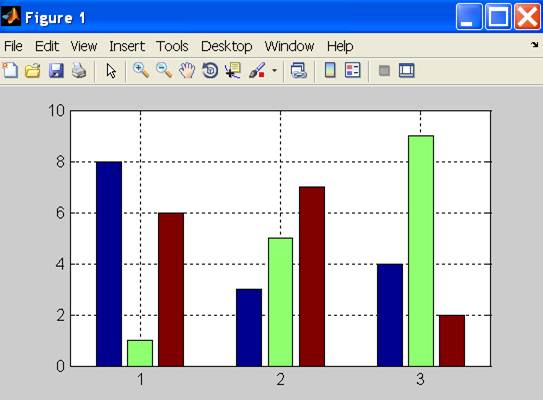### Save a Plot with print

• To add a legend, either use the legend command or use the insert command in the Menu Bar on the figure. Many other actions are available in Tools.
• It is convenient to use the Menu Bar to change a figure’s properties interactively. However, the set command is handy for non-interactive changes, as in an m-file.
• Similarly, save a graph via the Menu Bar’s File/’Save as’ or
``>> print -djpeg 'mybar'      % file mybar.jpg saved in current dir``

### Use MATLAB Command Syntax or Function Syntax?

Many MATLAB utilities are available in both command and function forms.

For this example, both forms produce the same effect:

``````>> print  -djpeg  'mybar'     % print as a command
>> print('-djpeg', 'mybar')   % print as a function``````

For this example, the command form yields an unintentional outcome:

``````>> myfile = 'mybar';          % myfile is defined as a string
>> print -djpeg    myfile     % as a command, myfile is 'myfile' (verbatim), not 'mybar'
>> print('-djpeg', myfile)    % as a function, myfile is treated as a variable``````

Other frequently used utilities that are available in both forms are `save` and `load`

### Surface Plot

``````>> Z = peaks;   % generate data for plot
>> surf(Z)      % surface plot of Z``````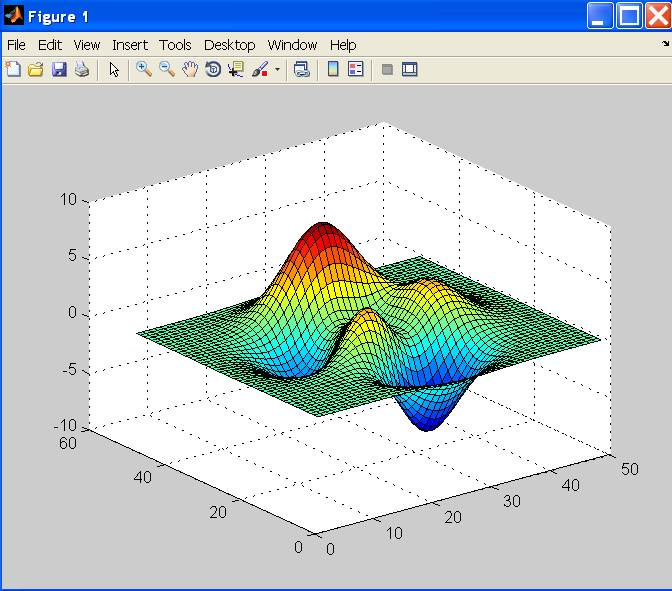Try these commands to see their effects:

``````>> shading flat
>> grid off
>> axis off
>> colorbar
>> colormap('winter')
>> colormap('jet')``````

### Contour Plots

``````>> Z = peaks;
>> contour(Z, 20)      % contour plot of Z with 20 contours````````````>> contourf(Z, 20);    % with color fill
>> colormap('hot')     % map option
>> colorbar            % make color bar``````### Integration Example

For a more practical example, we turn to numerical integration. While there are many integration techniques that are more efficient, we will use a mid-point integration for its simplicity. Lets consider the integration of cosine from 0 to π/2. In the following figure, 8 discrete increments are used for the numerical integration. In reality, of course, the number will be higher to get a reasonably accurate result. With a mid-point rule, the integrand is assumed to be constant within the increment (see the equation below).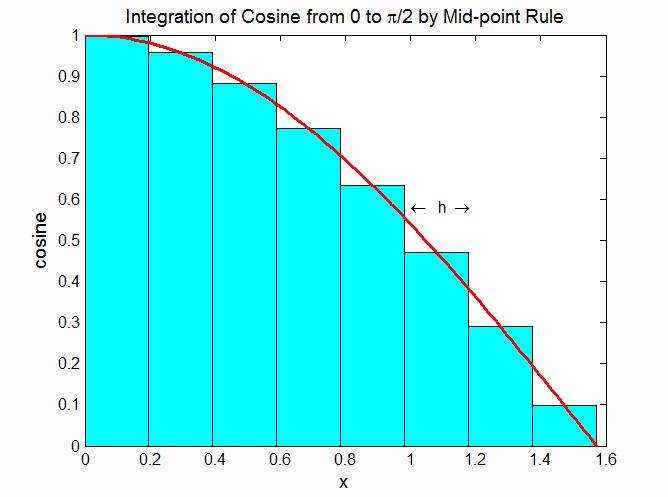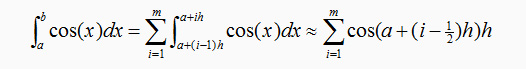In the above, a, b are, respectively, the lower and upper limits of integration while m is the number of increments. The increment, h, is determined by h = (b – a)/m.
``````% Integration with for-loop
tic
m = 100;             % number of increments
a = 0;               % lower limit of integration
b = pi/2;            % upper limit of integration
h = (b - a)/m;       % increment length
integral = 0;        % initialize integral
for i=1:m
x = a+(i-0.5)*h;   % mid-point of increment i
integral = integral + cos(x)*h;
end
toc````````````% Integration with vector form
tic
m = 100;             % number of increments
a = 0;               % lower limit of integration
b = pi/2;            % upper limit of integration
h = (b - a)/m;       % increment length
x = a+h/2:h:b-h/2;   % mid-point of m increments
integral = sum(cos(x)*h);
toc``````
In practically all cases, computations expressed with the vector form are significantly more efficient than its for-loop counterpart.

### Hands On Exercise

• Use the editor to write a program to generate the figure that describes the integration scheme we discussed. (Hint: use `plot` to plot the cosine curve. Use `bar` to draw the rectangles that depict the integrated value for each increment.) Save as `plotIntegral.m`
• Compute the integrals using 10 different increment sizes (h), for m=10, 20, 30, . . . , 100. Plot these 10 values to see how the solution converges to the analytical value of 1.

### Hands On Exercise Solution

``````a = 0;  b=pi/2;            % lower and upper limits of integration
m = 8;                     % number of increments
h = (b-a)/m;               % increment size
x= a+h/2:h:b-h/2;          % m mid-points
bh = bar(x,cos(x),1,'c');  % make bar chart with bars in cyan
hold                       % all plots superimposed on same figure
x = a:h/10:b;              % use more points to evaluate cosine
f = cos(x);                % compute cosine at x
ph = plot(x,f,'r');        % plots x vs f, in red
% Compute integral with multiple m to study convergence
for i=1:10
n(i) = 10+(i-1)*10;
integral(i) = sum(cos(x)*h);
end
figure   % create a new figure
plot(n, integral)``````

### More Hands On Exercises

• How would you generalize the script for arbitrary a & b ?
• How to add a title ?
• How about x-, and y-label ?

### Where Can I Run MATLAB ?

There are a number of ways: